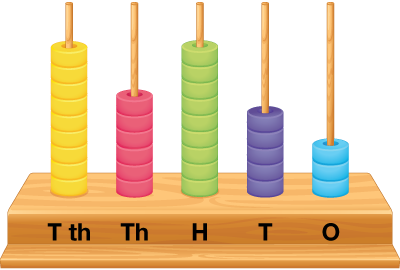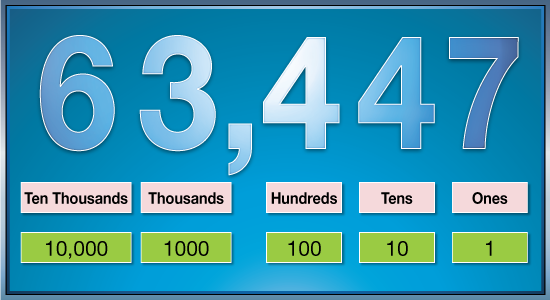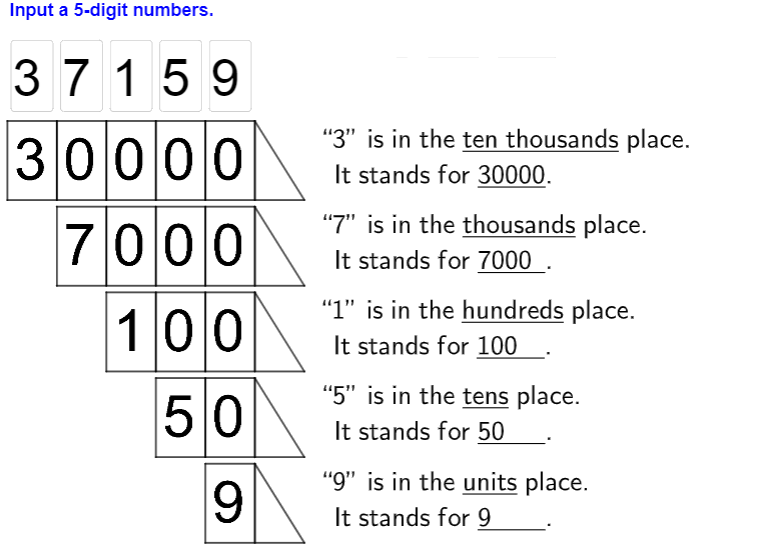# Numbers up to 5-Digits

Go back to  'Numbers and Place values'

## Introduction to Numbers up to $$5$$-Digits

When we talk of $$5$$-digit numbers, we talk of place values up to $$10000,$$ which can be represented thus:The place value of the five digits that make up the $$5$$-digit number would be obtained by progressively multiplying the respective figure by $$1, 10, 100, 1000$$ and $$10000,$$ starting from the rightmost digit and moving left.

## The Big Idea: Numbers up to $$5$$-Digits

### A simple idea: The Place Value of Numbers

As shown above, in a $$5$$-digit number, every progressive place value is the earlier place value multiplied by $$10.$$Thus we see that $$5$$ digit numbers show the value of the range $$10000.$$ The smallest $$5$$-digit number is $$10000,$$ while the largest $$5$$-digit number is $$99999.$$ These $$90000$$ possible $$5$$-digit numbers can have any number from $$0$$ to $$9$$ in any of the places, except for the placement of zero such that there is no non-zero number to its left. For example, $$20340$$ is a five digit number, but $$02340$$ is actually a $$4$$ digit number $$2340$$.### How To Decompose $$5$$-digit numbers

When we see a $$5$$-digit number, the five digits will correspond to the 5 place values units, tens, hundreds, thousands and ten thousands. Let us see how a $$5$$-digit number can be decomposed into its place values. Let us continue with the same number $$20340.$$

\begin{align} & { 2 \text { in Ten Thousand's place value } = 2 \times 10000 = 20000 } \\& { 0 \text { in Thousand's place value = } 0 \times 1000 = 0 } \\& { 3 \text { in Hundred's place value } = 3 \times 100 = 300 } \\& { 4 \text { in Ten's place value } = 4 \times 10 = 40 } \\& { 0 \text { in Unit's place value } = 0 \times 1 = 0 } \end{align}

So the five digit number can be expressed in the following way:

\begin{align} { 20000 } \\ { + \quad\, 0 } \\ { + \,300 } \\ { + \;\;\,40 } \\ { + \quad \; 0 } \\ \hline 20340 \\ \hline \;\; \end{align}

How would the $$5$$ place values change if we interchanged some of the digits? Let us say we have the $$5$$ digit number $$32004.$$

This the change in place value of the same $$5$$ digits:

• $$2$$ would now have place value of \begin{align} 2 \times 1000 = 2000 \end{align} (earlier place value of $$2$$ was $$20000$$)
• $$3$$ would now have place value of \begin{align} 3 \times 10000 = 30000 \end{align} (earlier place value of $$3$$ was $$300$$)
• $$4$$ would have place value of \begin{align} 4 \times 1 = 4 \end{align} (earlier place value was $$40$$)
• Zero is the only digit from $$0$$ to $$9$$ whose place value stays the same (zero) irrespective of its position. In both the above numbers, all the zero digits have zero place value.

### Commas in $$5$$-digit numbers

We saw the use of commas in four-digit numbers, and the position of the comma was to the left of the hundred's place. In a $$5$$-digit number too, only one comma is to be used, and it is to be applied to the left of the hundred's place. For example, the $$5$$-digit numbers $$32958$$ and $$20340$$ can be better expressed as $$32,958$$ and $$20,340$$ which helps in reading the numbers correctly.

## How is it important?

### How Many $$5$$-Digit Numbers can be formed

As we said earlier, $$02340$$ is not a five digit number but a four-digit number $$2340.$$ A five digit number cannot have $$0$$ as the first digit on the left, which is the ten thousand’s place.

Overall, every one of the $$5$$ places of a $$5$$-digit number can be filled up in ten ways, because it can have $$0, 1, 2, 3, 4, 5, 6, 7, 8,$$ or $$9.$$ So each of the five places can be similarly filled up in ten ways. Therefore, the total number of combinations possible are: \begin{align} 10 \times 10 \times 10 \times 10 \times 10 = 1,00,000 \end{align}

Out of these $$1,00,000$$ ways of forming a $$5$$-digit number, we need to take out all the possibilities which have zero in the first position on the left (or the ten thousand’s place). When there is zero in the first place, the next position (Thousand's) can be filled up in ten ways, as can the Hundred's, Ten's and Unit's places. So the number of $$5$$-digit numbers that have zero as the first digit are:

\begin{align}10 \times 10 \times 10 \times 10 = 10,000\end{align}

If we subtract these $$10,000$$ ways from the overall $$1,00,000$$ ways, we are left with $$90,000.$$

Therefore, there are $$90,000$$ unique $$5$$-digit numbers possible.